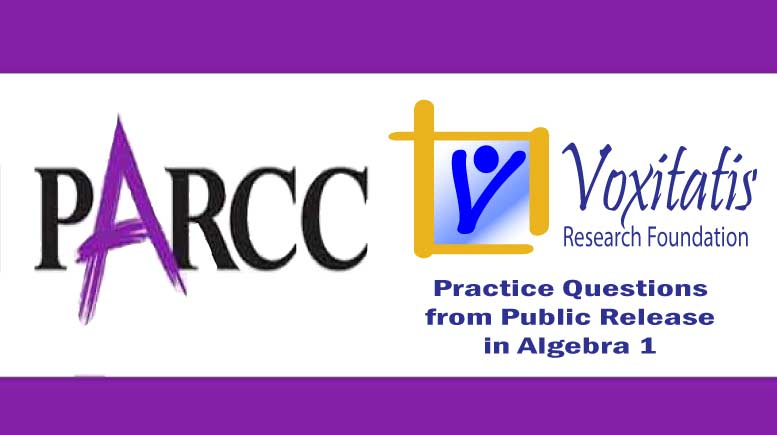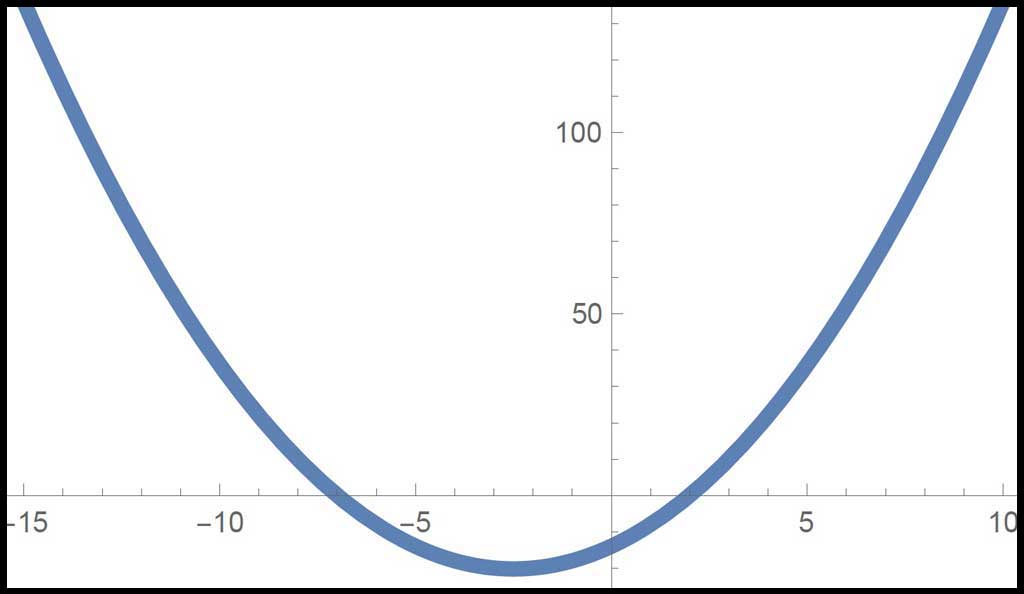Sunday, April 18, 2021# Algebra 1 PARCC question: quadratic zeros

-#### The following fill-in-the-blank question, explained here in hopes of helping algebra students in Maryland and Illinois prepare for the PARCC test near the end of this school year, appears on the released version of PARCC’s Spring 2015 test in algebra 1, here:

What are the solutions to the equation$(2x + 1)^2 - (x+13) = 3x^2 -2x + 2$ ?

To respond, fill in the blank boxes next to two “x = __” prompts in the equation editor.

Answer and references

Correct answers: –7 and 2.

PARCC evidence statement(s) tested: A.REI.4b-1:

Solve quadratic equations in one variable. … Solve quadratic equations with rational number coefficients by inspection (e.g., for x2 = 49), taking square roots, completing the square, the quadratic formula and factoring, as appropriate to the initial form of the equation.

i) Tasks should exhibit variety in initial forms. Examples of quadratic equations with real solutions: t2 = 49, 3a2 = 4, 7 = x2, r2 = 0, ½y2 = 1/5, y2 – 8y + 15 = 0, 2x2 – 16x + 30 = 0, 2p = p2 + 1, t2 = 4t, 7x2 + 5x – 3 = 0, ¾c(c – 1) = c, (3c − 2)2 = 6x − 4

ii) Methods are not explicitly assessed; strategy is assessed indirectly by presenting students with a variety of initial forms.

iii) For rational solutions, exact values are required. For irrational solutions, exact or decimal approximations may be required. Simplifying or rewriting radicals is not required; however, students will not be penalized if they simplify the radicals correctly.

iv) Prompts integrate mathematical practices by not indicating that the equation is quadratic. (e.g., “Find all real solutions of the equation t2 = 4t” … not, “Solve the quadratic equation t2 = 4t”).

The evidence statement above references Math Practice 5 and Math Practice 7 in the Common Core:  Mathematically proficient students consider the available tools when solving a mathematical problem. These tools might include pencil and paper, concrete models, a ruler, a protractor, a calculator, a spreadsheet, a computer algebra system, a statistical package, or dynamic geometry software. … For example, mathematically proficient high school students analyze graphs of functions and solutions generated using a graphing calculator. They detect possible errors by strategically using estimation and other mathematical knowledge. When making mathematical models, they know that technology can enable them to visualize the results of varying assumptions, explore consequences, and compare predictions with data. …

 Mathematically proficient students look closely to discern a pattern or structure. … Students will see 7 × 8 equals the well remembered 7 × 5 + 7 × 3, in preparation for learning about the distributive property. In the expression x2 + 9x + 14, older students can see the 14 as 2 × 7 and the 9 as 2 + 7. … They also can step back for an overview and shift perspective. They can see complicated things, such as some algebraic expressions, as single objects or as being composed of several objects. For example, they can see 5 – 3(x – y)2 as 5 minus a positive number times a square and use that to realize that its value cannot be more than 5 for any real numbers x and y.

The question tests students’ understanding of the high school Common Core algebra standard HSA.SSE.B.3, found under high school algebra (seeing structure in expressions), which states that they should be able to “choose and produce an equivalent form of an expression to reveal and explain properties of the quantity represented by the expression [and, 3.A] factor a quadratic expression to reveal the zeros of the function it defines.”

Example of a solution strategy (there are others)

Solve a quadratic equation for x (hopefully by factoring).$(2x + 1)^2 - (x+13) = 3x^2 -2x + 2$

I first notice the overall structure of the equation: I have x2, x, and a constant on each side, and so like terms can be combined easily. Squaring the binomial on the left, we get$4x^2 + 4x + 1 - x - 13 = 3x^2 - 2x + 2$

Combining like terms and moving everything over to the left side, we quickly get$x^2 + 5x - 14 = 0$

I can (thankfully) factor the left side by noting that –2 × 7 = –14 and –2 + 7 = 5.$(x-2)(x+7) = 0$

Once we get to this point, we know from the multiplicative property of 0 that if a × b = 0, then either a = 0, b = 0, or both a and b = 0.

So if (x–2) = 0, then x obviously is 2, and if (x+7) = 0, then x must be –7.

All solutions should be checked, which I can do in any number of ways. If I have a graphing calculator (or Mathematica) handy, I can plot the quadratic function on a coordinate grid and see where the graph intersects the x axis. It should be at the solutions I found for x.If I don’t have the means to graph the function, say during the PARCC test, I can plug the values I found for x back into the equation and make sure it’s true.$[2(-7) + 1]^2 - (-7+13) = 3(-7)^2 -2(-7) + 2$$(-13)^2 - 6 = (3)(49) - 2(-7) + 2$$169 - 6 = 147 + 14 + 2 \quad\textrm{check}$$[2(2) + 1]^2 - (2+13) = 3(2)^2 -2(2) + 2$$5^2 - 15 = (3)(4) - 2(2) + 2$$25 - 15 = 12 - 4 + 2 \quad\textrm{check}$

## Resources for further study

Purple Math, developed by Elizabeth Stapel, a math teacher from the St Louis area, has a six-part series on solving quadratic equations by factoring, as I did above. The series starts here.

The Khan Academy, developed by Sal Khan, an engineer who has created a library of thousands of video lessons, has a series of video lessons that demonstrate how to factor quadratics, starting here. He starts by factoring x2 – 14x + 40 as (x–4)(x–10), even though the text on his site says the second factor is (x–1). Despite the minor error in marketing, which would be unforgivable if done on the PARCC test, the video’s fine. Mr Khan clearly understands completely how to factor quadratics, even if he typed it incorrectly on his site.

Chapter 4, Section 4.4, of the book Algebra 2, Illinois edition by Ron Larson et al deals with solving quadratic equations by factoring. Students are trained to spot the structure in polynomial expressions of the general form$ax^2 + bx + c = (kx+m)(lx+n) = klx^2 + (kn+lm)x + mn$

where k and l must be factors of a and m and n must be factors of c.

Complete reference: Ron Larson, Laurie Boswell, Timothy D Kanold, Lee Stiff. Algebra 2, Illinois edition. Evanston, Ill.: McDougal Littell, a division of Houghton Mifflin Company, 2008. The book is used in several algebra classes taught in Illinois high schools.

## Analysis of this question and online accessibility

The question measures knowledge of the Common Core standard it purports to measure and tests students’ ability, by recognizing structure in an expression, to factor a quadratic equation to find the zeros. It is considered to have a median cognitive demand.

(The assertion in PARCC’s evidence statement that the math practices are assessed [integrate mathematical practices by not indicating that the equation is quadratic] is only weakly supported in that simply not telling the student the equation conforms to some vocabulary word [quadratic] fails to test the underlying mathematics. Furthermore, by not requiring or scoring students’ use of technology-based tools and other methods to check the solution, the PARCC item here fails to incorporate a significant part of Common Core Math Practice 5.)

The question can be tested online and should yield results that are as valid and reliable as those obtained on paper. Students online may experience difficulties with the equation editor if they don’t find the simple integer solutions in this case and try to type in a more complex albeit incorrect answer or answers. However, since the score on the item depends only on the correct answers, students aren’t penalized and don’t suffer from an inability to use the equation editor.

No special accommodation challenges can be identified with this question, so the question is considered fair.

## Challenge

Explain why, using the form of a two-column mathematical proof, the quadratic formula would yield exactly the same solutions as the factoring method used above. For reference the quadratic formula is$x = \frac{b \pm \sqrt{b^2 - 4ac}}{2a}$

where a is the coefficient of x2, b is the coefficient of x, and c is the constant in a quadratic equation of the form$ax^2 + bx + c = 0$

## Purpose of this series of posts

Voxitatis is developing blog posts that address every algebra 1 question released to the public by the Partnership for Assessment of Readiness for College and Careers, or PARCC, in order to help students prepare to take the test this spring.

Our total release will run from February 27 through March 15, with one or two questions discussed per day. Then we’ll move to geometry at the end of March, algebra 2 during the first half of April, and eighth grade during the last half of April.Paul Katulahttps://news.schoolsdo.org
Paul Katula is the executive editor of the Voxitatis Research Foundation, which publishes this blog. For more information, see the About page.

### Md. to administer tests in math, English

0
Students in Md. will still have to take standardized tests this spring in math and English language arts, following action of the state board.